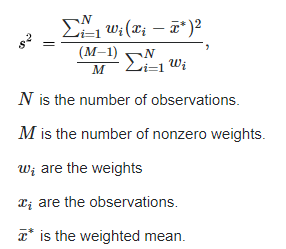Altair® Panopticon

### Weighted Sample Standard Deviation and Weighted Sample Variance

The formula used for calculation of the weighted sample Standard Deviation (“Weighted Stdev”) and weighted sample Variance (“Weighted Variance”) is the following, defined by NIST.gov, National Institute of Standards and Technology:Example with sample data:

 Value 6 7 8 9 10 11 12 23 Weight 1 1 1 1 1 1 1 100

 Mean Weighted Mean Sample Standard Deviation Weighted sample Standard Deviation Sample Variance Weighted sample Variance 10.75 22.08 5.34 3.74 28.50 13.99